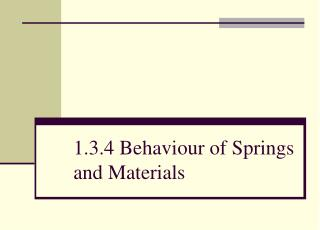DownloadDownload Presentation1.3.4 Behaviour of Springs and Materials

# 1.3.4 Behaviour of Springs and Materials

Télécharger la présentation## 1.3.4 Behaviour of Springs and Materials

- - - - - - - - - - - - - - - - - - - - - - - - - - - E N D - - - - - - - - - - - - - - - - - - - - - - - - - - -
##### Presentation Transcript

1. 1.3.4 Behaviour of Springs and Materials

2. Objective Describe how deformation is caused by a force in one direction and can be tensile or comprehensive

3. Deformation Can be caused by tensile or compressive forces • Tensile • cause tension • stretching forces • Compressive • cause compression • squeezing forces

4. Deformation two equal and opposite tensile forces stretching a wire two equal and opposite compressive forces squeezing a spring

5. Objective Describe the behaviour of springs and wires in terms of force, extension, elasticlimit, Hooke’s Law and the force constant – i.e. force per unit extension or compression

6. Definitions • Force (F) • applied to a spring or wire in tension or compression • Extension (x) • the change in length of a material when subjected to a tension, measured in metres • Elastic Limit • the point at which elastic deformation becomes plastic deformation

7. Definitions • Elastic Deformation • when the deforming force is removed, the object will return to it’s original shape • eg rubber band, spring (usually) • Plastic Deformation • when the deforming force is removed, the object will not return to it’s original shape • eg Plasticine, Blutack

8. Hooke’s Law • When tension is plotted against extension, a straight line graph denotes elastic deformation • This is summarised by Hooke’s Law: ‘The extension of a body is proportional to the force that causes it’ or as a formula: where F = Force F = kx x = extension k = force/spring constant

9. Hooke’s Law F Tension /N x Extension /mm

10. Force/Spring Constant • F = kx • Expressed in newtons per metre • How much force is required per unit of extension • eg 5 N mm-1 means a force of 5 N causes an extension of 1 mm • Can only be used when the material is undergoing elastic deformation

11. Objective Determine the area under a force against extension (or compression) graph to find the work done by the force

12. Work Done • Extension produced by tension F is x • Work done to reach this extension is the area under the graph work done = area of triangle = ½Fx

13. Objective Select and use the equations for elastic potential energy, E = ½Fx and ½kx2

14. Elastic Potential Energy • As work has been done to stretch the wire, the wire then stores Elastic Potential Energy • This also applies to compression forces • For elastic deformation, the elastic potential energy equals work done: E = ½Fx as F = kx then E = ½kx2

15. Objective Define and use the terms stress, strain, Young modulus and ultimate tensile strength (breaking stress)

16. Stretching Materials • One way of describing the property of a material is to compare stiffness • In order to calculate stiffness, two measurements need to be made: • strain • stress

17. Stretching Materials • Strain is the fractional increase in the length of a material Strain = extension (m) original length (m) • Stress is the load per unit cross-sectional area of the material Stress (Nm-2) = force (N) cross-sectional area (m2)

18. Young Modulus • To calculate stiffness, calculate the ratio of stress to strain: Young Modulus (Nm-2) = stress strain or E = stress strain

19. Young Modulus Hooke’s Law Region Elastic limit Limit of proportionality stress gradient = Young modulus strain

20. Ultimate Tensile Stress • Stiffness tells us about the elastic behaviour of a material (Young modulus) • Strength tells us how much stress is needed to break the material • The amount of stress supplied at the point at which the material breaks is called the ultimate tensile stress of the material

21. Objective Describe an experiment to determine the Young modulus of a metal in the form of a wire

22. Young Modulus Practical Young modulus practical

23. Objective Define the terms elastic deformation and plastic deformation of a material

24. Definitions • Elastic Deformation • when the deforming force is removed, the object will return to it’s original shape • eg rubber band, spring (usually) • Plastic Deformation • when the deforming force is removed, the object will not return to it’s original shape • eg Plasticine, Blutack

25. Objective Describe the shapes of the stress against strain graphs for typical ductile, brittle and polymeric materials

26. Ductile • Will stretch beyond it’s elastic limit • Will deform plastically • Can be shaped by stretching, hammering, rolling and squashing • Examples include copper, gold and pure iron

27. Brittle • Will not stretch beyond it’s elastic limit • Will deform elastically • Will shatter if you apply a large stress • Examples include glass and cast iron

28. Polymeric Will perform differently depending on the molecular structure and temperature • Can stretch beyond it’s elastic limit • Can deform plastically • Can be shaped by stretching, hammering, rolling and squashing • Examples include polythene

29. Polymeric • Cannot stretch beyond it’s elastic limit • Can deform elastically • Can shatter if you apply a large stress • Examples include perspex

30. Summary • All materials show elastic behaviour up to the elastic limit • Brittle materials break at the elastic limit • Ductile materials become permanently deformed beyond the elastic limit • Polymeric materials can show either characteristics, depending on the molecular structure and temperature

31. Questions • Physics 1 – Chapter 8 • SAQ 1 to 9 • End of Chapter questions 1 to 4